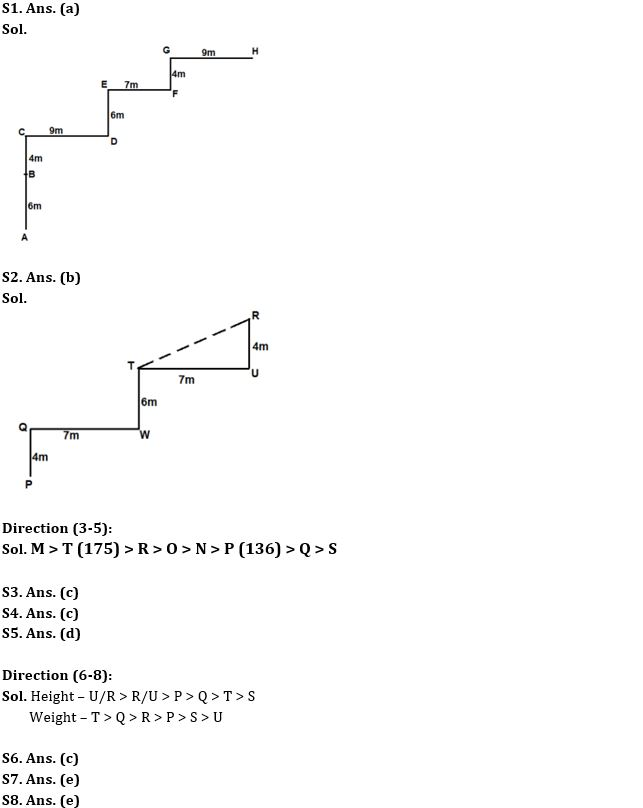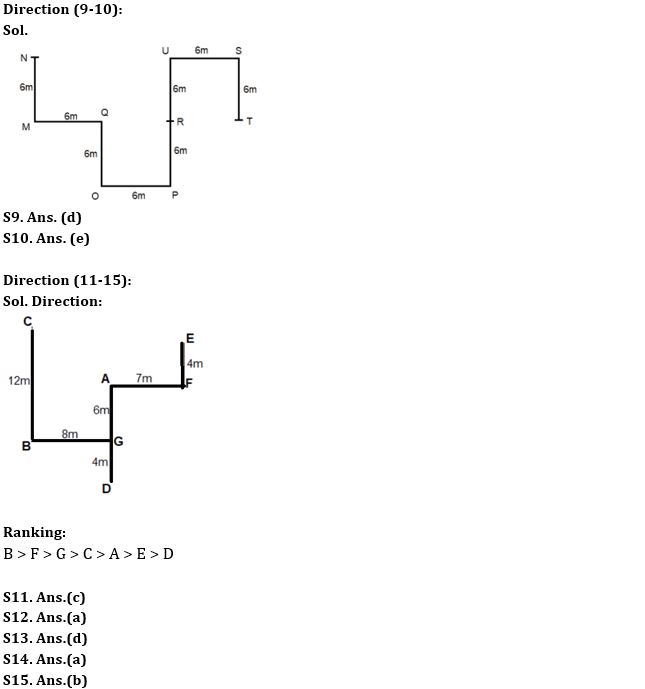Latest Banking jobs   »

# Reasoning Ability Quiz For IBPS RRB PO Clerk Mains 2022- 1st September

Directions (1-2): Answer the questions based on the information given below.
In coded language,
X&Y means Y is 6m north of X.
X\$Y means X is 4m south of Y.
X#Y means Y is 9m east of X.
X@Y means X is 7m west of Y.

Q1. In expression A&B\$C#D&E@F\$G#H, what is the direction of H with respect of A?
(a) North-east
(b) North-west
(c) East
(d) South-east
(e) None of the above

Q2. In expression P\$Q@W&T@U\$R, what is the shortest distance between T and R?
(a) √85m
(b) √65m
(c) √75m
(d) 8m
(e) None of the above

Directions (3-5): Answer the questions based on the information given below.
Eight persons P, M, N, O, Q, R, S, and T have different heights. O is % P who is % Q. R is % P but @ M. O is @ R. S is @ Q and N is @ O but % P. T is @ M but % R. Height of the one who is second tallest is 175 cm and the one who is third shortest is 136 cm.
Note – % means ‘taller than’ and @ means ‘shorter than’

Q3. If O’s height is 170m then what can be the possible height of R?
(a) 176cm
(b) 179m
(c) 172cm
(d) 177cm
(e) None of the above

Q4. How many persons are shorter than M but taller than Q?
(a) Three
(b) Four
(c) Five
(d) Six
(e) None of the above

Q5. What can be the possible height of S?
(a) 138cm
(b) 140m
(c) 142cm
(d) 124cm
(e) None of the above

Directions (6-8): Answer the questions based on the information given below.
Six persons P, Q, R, S, T and U have different heights and weights. No two persons have same rank for height and weight i.e., if one person is tallest then he/she cannot be heaviest. R is heavier and taller than P. Q is taller than only T and S. S is heavier than only U. T is heavier than Q who is heavier than R. U is taller than P.

Q6. Who among the following is the heaviest?
(a) U
(b) P
(c) T
(d) Q
(e) R

Q7. How many persons are shorter than R?
(a) Two
(b) Three
(c) Five
(d) Four
(e) Cannot be determined

Q8. Who among the following is the shortest?
(a) U
(b) P
(c) T
(d) Q
(e) S

Directions (9-10): Answer the questions based on the information given below.
If D? E means D is 6m east of E
D+E means D is 6m west of E
D*E means D is 6m north of E
D%E means D is 6m south of E

Given expression – N*M+Q*O+P%R %U+S*T

Q9. In which direction is point T with respect to point N?
(a) North-east
(b) North-west
(c) South-west
(d) South-east
(e) None of the above

Q10. Four among the following five are same in a certain way and related to a group. Which of the following does not belong to the group?
(a) N, M
(b) U, R
(c) S, T
(d) R, P
(e) S, Q

Directions (11-15): Answer the questions based on the information given below:
Seven players A, B, C, D, E, F and G score different runs in a match. Each of them is standing randomly in the playground.
The only player who scores less than E, is 4m south of G. Player who scores the 3rd lowest run is 7m west of the one who scores more than G and C. One of the players is 8m west of G. A is 10m north of D who scores the lowest runs. F is not the highest scorer. C doesn’t score the 3d highest runs. More than three players score more than the one who is 6m north of G. E is 4m north of F. C is 12m north of the highest scorer who is 10m southwest of A.

Q11. What is the direction of the highest run scorer with respect to F?
(a) North-east
(b) North-west
(c) South-west
(d) South-east
(e) None of the above

Q12. Which among the following is to the north-west of the person who scores third highest runs?
(a) C
(b) E
(c) B
(d) D
(e) None of the above

Q13. What is the shortest distance between B and the player who is to the west of F?
(a) 15m
(b) 13m
(c) 20m
(d) 10m
(e) None of the above

Q14. How many players score more than one who is to the east of B?
(a) Two
(b) Three
(c) One
(d) Four
(e) None of the above

Q15. What is the direction of C with respect to D?
(a) North-east
(b) North-west
(c) South-west
(d) South-east
(e) None of the above

Solutions#### Congratulations!Download Hindu Review of October 2021: Free PDF